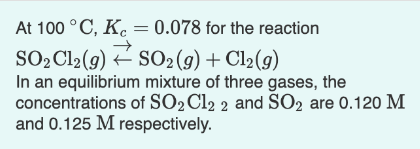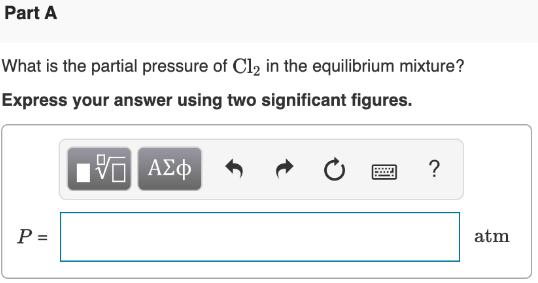# At 100 °C, Kc = 0.078 for the reaction SO2Cl2(g) ⇌ SO2(g) + Cl2(g) In an equilibrium mixture of three gases, the concentrations of SO2Cl2 2 and SO2 are 0.120 M and 0.125 M respectively. What is the partial pressure of Cl2 in the equilibrium mixture? Express your answer using two significant figures.